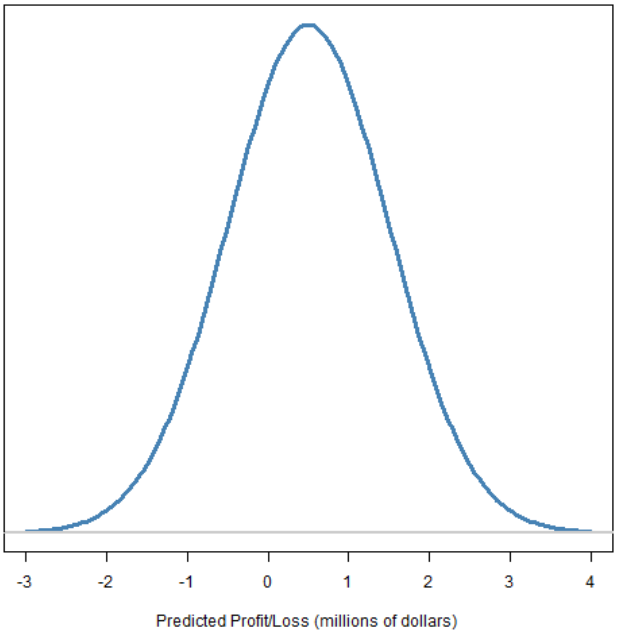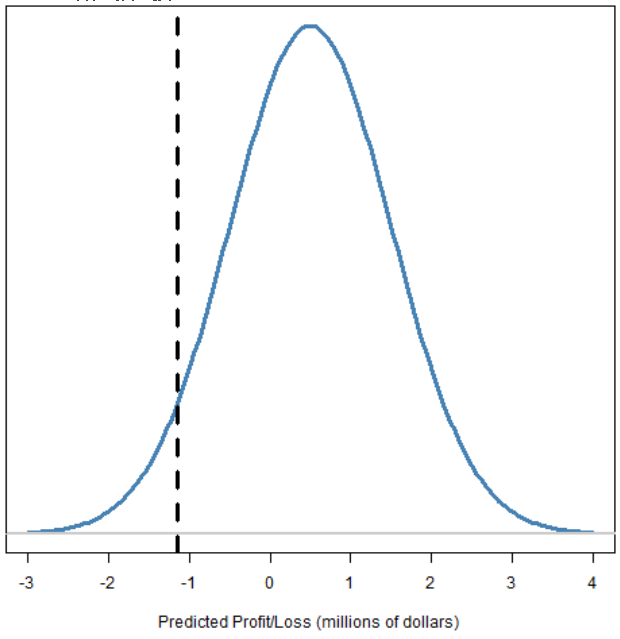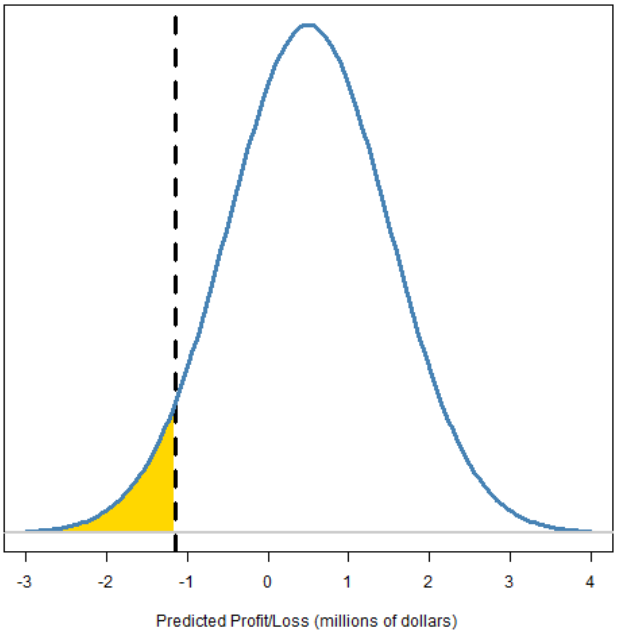# R语言风险价值VaR（Value at Risk）和损失期望值ES（Expected shortfall）的估计

## 金融风险管理:VaR 和 ES 的度量• 条件风险价值（CVaR）
• 平均短缺
• 平均超额损失

## 缩略语

“风险价值”的缩写有可能与其他两个概念混淆：

• 方差
• 向量自回归

• VaR：风险价值
• var：方差
• VAR：向量自回归

## 估算

• 投资组合中的资产
• 所涉及资产的价格历史

### 衍生成分

• 拟合假设分布
• 模拟（使用一段时间内的经验分布）
• 总体预测
• 梯度模拟

## R语言

### 填充区域


plot(xseq, pd, type="l", col="steelblue", lwd=3,
yaxt="n", ylab="",
xlab="Predicted Profit/Loss (millions of dollars)")

abline(v=qnorm(.05, mean=.5, sd=1), lty=2, lwd=3)

polygon(c(xseqt, max(xseqt)), c(dnorm(xseqt,
mean=.5, sd=1), 0), col="gold", border=NA)

lines(xseq, pd, type="l", col="steelblue", lwd=3)
abline(h=0, col="gray80", lwd=2)# R语言基于ARMA-GARCH-VaR模型拟合和预测实证研究分析案例

### 投资组合方差计算

weight %*% varianceMatrix %*% weight


weight %*% varianceMatrix[names(weight),
names(weight)] %*% weight

# 风险价值（VaR）和预期短缺（ES）始终与投资组合有关。

• 投资组合
• 所涉及资产的价格历史

## 单变量估计

### 随时关注您喜欢的主题

R1 <- assetSimpRetMatrix %*% portWts


R1 <- assetSimpRetMatrix[, names(portWts)] %*% portWts


R1上面计算的对象持有投资组合的（假设的）简单收益。

r1 <- log(R1 + 1)


• 历史的（使用最近一段时间内的经验分布）
• 正态分布（根据数据估算参数）并使用适当的分位数
• t分布（通常假设自由度而不是估计自由度）
• 拟合单变量garch模型并提前进行模拟

### R分析

>  "historical")
[,1]
VaR -0.02515786
> "gaussian")
[,1]
VaR -0.0241509
>  "gaussian"
[,1]
VaR -0.03415703
>  "historical")
[,1]
ES -0.03610873
>  "gaussian")
[,1]
ES -0.03028617

no weights passed in, assuming equal weighted portfolio
$MVaR [,1] [1,] 0.02209855$contribution
Convertible Arbitrage            CTA Global
0.0052630876         -0.0001503125
Distressed Securities      Emerging Markets
0.0047567783          0.0109935244
Equity Market Neutral
0.0012354711

\$pct_contrib_MVaR
Convertible Arbitrage            CTA Global
0.238164397          -0.006801916
Distressed Securities      Emerging Markets
0.215252972           0.497477204
Equity Market Neutral
0.055907342

# 这是用于风险价值的历史估计的简单函数的定义：

### 风险价值的历史估计

VaRhistorical <- function(returnVector, prob=.05,
notional=1, digits=2)
{
if(prob > .5) prob <- 1 - prob
ans <- -quantile(returnVector, prob) * notional
signif(ans, digits=digits)
}

投资组合，例如：

> VaRhistorical(spxret11, notional=13e6)
5%
330000

EShistorical <- function(returnVector, prob=.05,
notional=1, digits=2)
{


> EShistorical(spxret11, notional=13e6)
 470000


### 正态分布

> VaRnormalEqwt(spxret11, notional=13e6)
 310000
> VaRnormalEqwt(spxret11, notional=13e6,
+     expected.return=0)
 310000

ESnormalEqwt <- function(returnVector, prob=.05,
notional=1, expected.return=mean(returnVector),
digits=2)
{

ans <- -tailExp * notional
signif(ans, digits=digits)


> ESnormalEqwt(spxret11, notional=13e6)
 390000

VaRnormalExpsmo <- function(returnVector, prob=.05,
notional=1, expected.return=mean(returnVector),
lambda=.97, digits=2)
{

signif(ans, digits=digits)


# 一个更好的办法是用指数平滑得到的波动性：

> VaRnormalExpsmo(spxret11, notional=13e6)
 340000

### t分布

VaRtExpsmo <- function(returnVector, prob=.05,
notional=1, lambda=.97, df=7, digits=2)
{
if(prob > .5) prob <- 1 - prob



> VaRtExpsmo(spxret11, notional=13e6)
2011-12-30
340000

Kaizong Ye拓端研究室（TRL）的研究员。

​非常感谢您阅读本文，如需帮助请联系我们！QQ在线咨询

15121130882

0571-63341498

## 关注有关新文章的微信公众号

This will close in 0 seconds